> > The Parallel Plate Capacitor

# The Parallel Plate Capacitor

You have heard us mention parallel plate capacitors previously. But, do you know what those are actually? Is it a setup that has two plates attached parallel to each other? Why don’t you find out the answer yourself! Read this chapter to know the concept of the parallel plate capacitor.

### Suggested VideosElectrostatic ForceElectric field at a point along the axis of Electric DipoleElectrostatic Force## The Parallel Plate Capacitor

Parallel Plate Capacitors are the type of capacitors which that have an arrangement of electrodes and insulating material (dielectric). The two conducting plates act as electrodes. There is a dielectric between them. This acts as a separator for the plates.

The two plates of parallel plate capacitor are of equal dimensions. They are connected to the power supply. The plate, connected to the positive terminal of the battery, acquires a positive charge. On the other hand, the plate, connected to the negative terminal of battery acquires a negative charge. Due to the attraction charges are in a way trapped within the plates of the capacitor.

## The Principle of Parallel Plate Capacitor

We know that we can give a certain amount of charge to a plate. If we supply more charge, the potential increases and it could lead to a leakage in the charge. If we get another plate and place it next to this positively charged plate, then negative charge flows towards the side of this plate which is closer to the positively charged plate.

As both the plates have charges, the negative charge on plate 2 will reduce the potential difference on plate 1. On the other hand, the positive charge on plate 2 will increase the potential difference on plate 1. But the negative charge on plate 2 will have more impact. So, more charge can be given on plate 1. Because of the negative charges on plate 2 the potential difference will be less. This is the principle of the parallel plate capacitor.

## Dependence of Charge Stored in a Capacitor

The amount of electric charge stored in any of the plates of parallel plate capacitor is directly proportional to the potential difference between the two plates of Parallel Plate Capacitor. This relation can be seen as:

Q $$\propto$$  V

Therefore, Q = (constant)×V = CV

Where C = Capacitance of the capacitor; Q = Amount of charge stored in one capacitor; V = Potential difference between the two plates.

### The Capacitance of Parallel Plate Capacitor

The capacitance of the parallel plate capacitor determines the amount of charge that it can hold. If you see the above equation, you will see that greater the value of C, greater will be the charge that a capacitor can hold. Therefore we can see that the capacitance depends upon:

• The distance d between two plates.
• The area A of the medium between the plates.

According to the Gauss law, we can write the electric field as: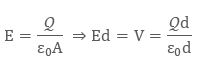Since we know that the capacitance is defined as V = Q/C, so we can write capacitance as:When the plates are placed very close and the area of plates are large, we get the maximum capacitance.

## Dielectric Material Inserted between Two Plates

On the two plates, the microscopic dipole moment of the material will shield the charges. Thus, it will alter the effect of dielectric material, inserted between the two plates. Materials have a permeability which is given by the relative permeability k. The capacitance is thus: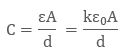We can increase the capacitance of a parallel plate capacitor by introducing a dielectric between the plates as the dielectric have permeability k, which is greater than 1. K is also sometimes known as Dielectric Constant.

### The condition of parallel plate capacitor when the medium is in the air and in other substance

When in parallel plate capacitor, the area between the who plates are partially filled with air and partially with other substance, its Capacitance can be calculated. Let there exist a parallel plate capacitor in which medium between the parallel plates is mainly the air and partially other substance as shown in the figure below: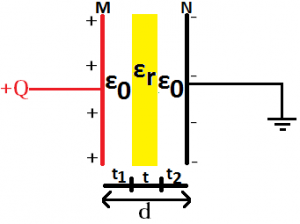## Multiple Parallel Plate Capacitor

The arrangement of parallel plate capacitor with dielectric material between them in groups fitting in each other is known as Multiple Parallel Plate Capacitor. The capacitance of multiple parallel plate capacitor can be calculated as: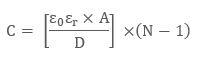where A = Area of each plate; ε0 = Relative Permittivity of a Vacuum = 8.854 × 10-12 F/m; εr = Relative Permittivity of Dielectric; D = Distance between plates; N = Number of Plates.

## Charge on Parallel Plate Capacitor

Let us assume that a capacitor has capacitance C and have electric charge Q and the capacitor is electrically neutral.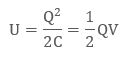Where V is the potential difference between the plates. Now if the charge upon the two plates of parallel plate capacitor is different then, V1 will be the potential difference of plate 1 with Q1 be the charge. While V2 will be the potential difference of plate 2 with charge Q2 = −Q + δQ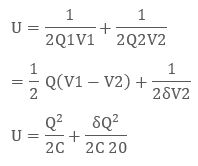## Solved Example for You

Q1: Assertion: The total charge stored in a capacitor is zero.
Reason: The field just outside the capacitor is σ/ε0. (σ is the charge density)

1. Both the statements are true and the reason is the correct explanation of the assertion.
2. The assertion and reason are true but the reason is not the correct explanation of the assertion.
3. Assertion is true, the reason is false.
4. The reason and the assertion are false.

Solution: C) The net charge on either plate of the capacitor is equal and opposite. So the net charge stored in a capacitor is = zero. The assertion is indeed correct. However if you can imagine a surface enclosing the plates of the capacitor, this surface will not hold any net charge and according to Gauss Theorem, the flux will be zero. Thus the field is zero outside the capacitor. Hence, the reason is false.

Share with friends

## Customize your course in 30 seconds

##### Which class are you in?
5th
6th
7th
8th
9th
10th
11th
12th
Get ready for all-new Live Classes!
Now learn Live with India's best teachers. Join courses with the best schedule and enjoy fun and interactive classes.Ashhar Firdausi
IIT Roorkee
BiologyDr. Nazma Shaik
VTU
ChemistryGaurav Tiwari
APJAKTU
Physics
Get Started

## Browse

##### Electrostatic Potential and CapacitanceSubscribe
Notify of

## Question Mark?

Have a doubt at 3 am? Our experts are available 24x7. Connect with a tutor instantly and get your concepts cleared in less than 3 steps.